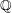# Algebraic independence of pi and e

 Importance: High ✭✭✭
 Author(s):
 Subject: Number Theory
 Keywords: algebraic independence
 Posted by: porton on: July 8th, 2008
Conjectureandare algebraically independent

## Bibliography

* indicates original appearance(s) of problem.

### Schanuel's conjecture

Assuming Schanuel's conjecture, one can show thatandare algebraically independent over.

### in which subfield K of which field L?

After all, e to the pi i = -1, so this shows that pi and e are not always algebraically independent.

### By definition?

I think any two distinct transcendental numbers must be algebraically independent, almost by definition. Since e and pi are transcendental, they must be a. i. No? - David Spector

### not all transcedentials are algebraically independant

pi and 4-pi are both transcedential and sum to 4, so are not algebraically independant.

### two transcendentals are not necessarily algebraically independen

e and e^2 are both transcendental but (e,e^2) makes the two-variable polynomial f(x,y)=x^2-y equal to zero

### ?

but that's not a polynomial.